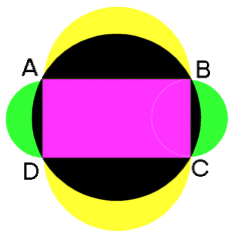#### You may also like### Some(?) of the Parts

A circle touches the lines OA, OB and AB where OA and OB are perpendicular. Show that the diameter of the circle is equal to the perimeter of the triangle### Polycircles

Show that for any triangle it is always possible to construct 3 touching circles with centres at the vertices. Is it possible to construct touching circles centred at the vertices of any polygon?### Circumspection

M is any point on the line AB. Squares of side length AM and MB are constructed and their circumcircles intersect at P (and M). Prove that the lines AD and BE produced pass through P.

# The Pillar of Chios

##### Age 14 to 16 Challenge Level:The whole shape is made up of a rectangle, two semicircles on $AB$ and on $DC$ together making one circle, and the two semicircles on $AD$ and $BC$ making another circle.

Excellent solutions were sent in by a pupil from Dr Challoner's Grammar School, Amersham and Nisha Doshi ,Y9, The Mount School, York. Here is one of their solutions: Take: $AB=2x, AD=2y$.
\begin{eqnarray} \mbox{Total area of shape} &=& \pi x^2 + \pi y^2 + (2x \times\ 2y)\\ &=& \pi x^2 + \pi y^2 + 4xy. \end{eqnarray}
By Pythagoras Theorem
\begin{eqnarray} AC^2 &=& AD^2 + DC^2\\ &=& (2x)^2 +\ (2y)^2\\ &=& 4(x^2 + y^2)\\ \mbox{Area of big circle} &=& \pi(\mbox{AC}/2)^2\\ &=& \pi(x^2 + y^2)\\ \mbox{Area of crescents} &=& \mbox{Area of shape - Area of big circle}\\ &=& \pi x^2 + \pi y^2 + 4xy - \pi (x^2 + y^2)\\ &=& 4xy\\ \mbox{Area of rectangle} &=& 2x \times\ 2y\\ &=& 4xy \end{eqnarray}
so the sum of the areas of the four crescents is equal in area to the rectangle $ABCD$.